# 1491 How Many Square Units Do You See in This Partially Completed Puzzle?

### Today’s Puzzle:

My grandchildren had put this puzzle together many times on their own but wanted me to help them this time. The picture on the box was very helpful and let me know there were four unicorns, but I could only find puzzle pieces for three horns instead of four. I found the missing horn under some furniture in the living room, but I still wondered if any other puzzle pieces had been misplaced. I used multiplication, addition, and subtraction to figure out how many puzzle pieces still needed to be placed on this partially completed puzzle. Can you also figure out the number of puzzle pieces that still need to be placed?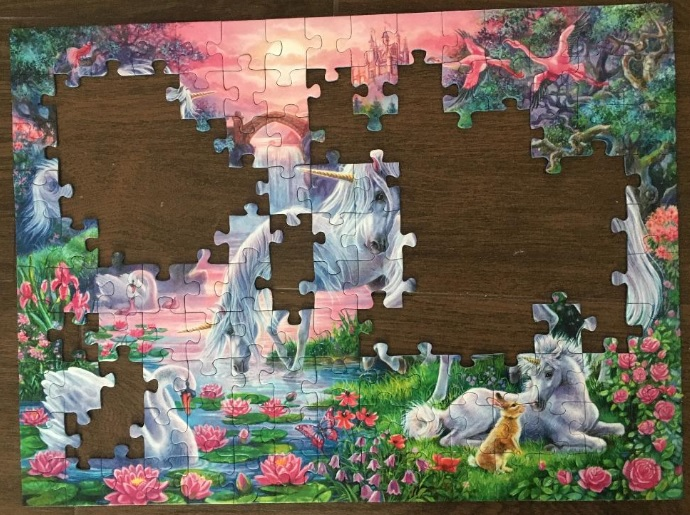I counted the number of puzzle pieces in the box and determined that two pieces were missing. Knowing that two puzzle pieces are missing, can you tell me how many more puzzle pieces are in the box waiting to be placed on the puzzle?

Each puzzle piece is approximately equal to one square unit. If you can determine the area of the entire puzzle and the area of the missing pieces, then you can determine the area of the incomplete puzzle pictured above. Go ahead, give it a try!

### Factors of 1491:

• 1491 is a composite number.
• Prime factorization: 1491 = 3 × 7 × 71.
• 1491 has no exponents greater than 1 in its prime factorization, so √1491 cannot be simplified.
• The exponents in the prime factorization are 1, 1, and 1. Adding one to each exponent and multiplying we get (1 + 1)(1 + 1)(1 + 1) = 2 × 2 × 2 = 8. Therefore 1491 has exactly 8 factors.
• The factors of 1491 are outlined with their factor pair partners in the graphic below.### Another Fact about the number 1491:

1491 is the 21st nonagonal number because
21(7·21 – 5)/2 = 1491.

# 1490 and Level 2

### Today’s Puzzle:

You can solve this puzzle if you know the basic multiplication and division facts. Just write the numbers from 1 to 12 in both the first column and the top row so the given clues and those factors create a valid multiplication table.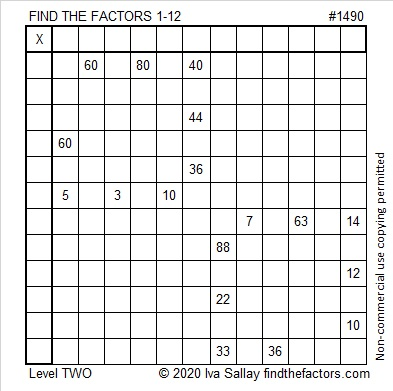### Factors of 1490:

• 1490 is a composite number.
• Prime factorization: 1490 = 2 × 5 × 149.
• 1490 has no exponents greater than 1 in its prime factorization, so √1490 cannot be simplified.
• The exponents in the prime factorization are 1, 1, and 1. Adding one to each exponent and multiplying we get (1 + 1)(1 + 1)(1 + 1) = 2 × 2 × 2 = 8. Therefore 1490 has exactly 8 factors.
• The factors of 1490 are outlined with their factor pair partners in the graphic below.### More Facts about the Number 1490:

1490 is the sum of two squares in two different ways:
31² + 23² = 1490.
37² + 11² = 1490.

1490 is the hypotenuse of FOUR Pythagorean triples:
432-1426-1490, calculated from 31² – 23², 2(31)(23), 31² + 23².
510-1400-1490, which is 10 times (51-140-149).
814-1248-1490, calculated from 2(37)(11), 37² – 11², 37² + 11².
894-1192-1490, which is (3-4-5) times 298.

# 1489 and Level 1

### Today’s Puzzle:

You can use the 20 clues in this puzzle to find the order that the factors from 1 to 12 must take. After you find those factors you can multiply each pair of factors together to complete the entire multiplication table.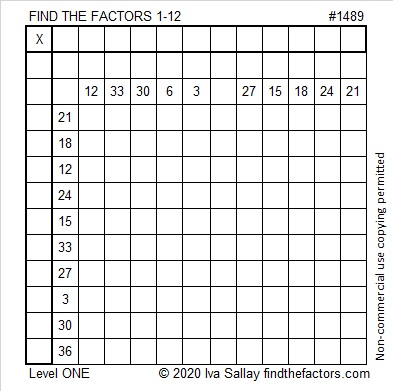### Factors of 1489:

• 1489 is a prime number.
• Prime factorization: 1489 is prime.
• 1489 has no exponents greater than 1 in its prime factorization, so √1489 cannot be simplified.
• The exponent in the prime factorization is 1. Adding one to that exponent we get (1 + 1) = 2. Therefore 1489 has exactly 2 factors.
• The factors of 1489 are outlined with their factor pair partners in the graphic below.How do we know that 1489 is a prime number? If 1489 were not a prime number, then it would be divisible by at least one prime number less than or equal to √1489. Since 1489 cannot be divided evenly by 2, 3, 5, 7, 11, 13, 17, 19, 23, 29 31, or 37, we know that 1489 is a prime number.

### Other Facts about the Number 1489:

1489 is the sum of two squares:
33² + 20² = 1489.

1489 is the hypotenuse of a Pythagorean triple:
689-1320-1489 calculated from 33² – 20², 2(33)(20), 33² + 20².

Here’s another way we know that 1489 is a prime number: Since its last two digits divided by 4 leave a remainder of 1, and 33² + 20² = 1489 with 33 and 20 having no common prime factors, 1489 will be prime unless it is divisible by a prime number Pythagorean triple hypotenuse less than or equal to √1489. Since 1489 is not divisible by 5, 13, 17, 29, or 37, we know that 1489 is a prime number.

1489 is the last prime number in the fifth prime decade: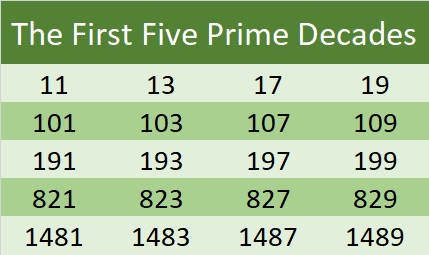1489 is a centered triangular number because (3(31²) + 3(31) + 2)/2 = 1489.# 1487 A Challenging Puzzle

### Today’s Puzzle:

This puzzle is like four out-of-order 1 – 10 multiplication tables that work together. The clues in the puzzle need to be the products of the numbers you write. It won’t be easy, but use logic to solve it. Good luck!Print the puzzles or type the solution in this excel file: 10 Factors 1478-1487

### Factors of 1487:

• 1487 is a prime number.
• Prime factorization: 1487 is prime.
• 1487 has no exponents greater than 1 in its prime factorization, so √1487 cannot be simplified.
• The exponent in the prime factorization is 1. Adding one to that exponent we get (1 + 1) = 2. Therefore 1487 has exactly 2 factors.
• The factors of 1487 are outlined with their factor pair partners in the graphic below.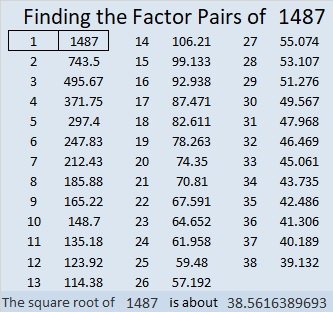How do we know that 1487 is a prime number? If 1487 were not a prime number, then it would be divisible by at least one prime number less than or equal to √1487. Since 1487 cannot be divided evenly by 2, 3, 5, 7, 11, 13, 17, 19, 23, 29 31, or 37, we know that 1487 is a prime number.

### Other Facts about the Number 1487:

1487 is the difference of two squares:
744²-743² = 1487.

1487 is the third prime number in the fifth prime decade. See it in the list below:# 1486 Mysterious Cat

### Today’s Puzzle:

If a cat has nine lives, how many lives do seven cats have? Where do the numbers 9 and 7 belong in this puzzle? Where do all the other numbers from 1 to 10 belong?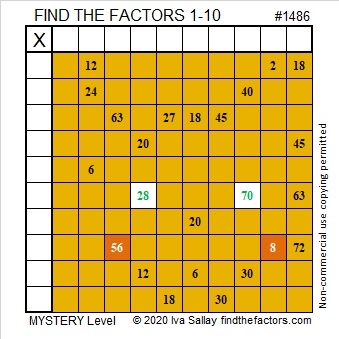### Factors of 1486:

• 1486 is a composite number.
• Prime factorization: 1486 = 2 × 743
• 1486 has no exponents greater than 1 in its prime factorization, so √1486 cannot be simplified.
• The exponents in the prime factorization are 1, and 1. Adding one to each exponent and multiplying we get (1 + 1)(1 + 1) = 2 × 2 = 4. Therefore 1486 has exactly 4 factors.
• The factors of 1486 are outlined with their factor pair partners in the graphic below.### More about the Number 1486:

1486 is not the sum of two squares or the difference of two squares, but it is in a Pythagorean triple:
1486-552048-552050, calculated from 2(743)(1), 743² – 1², 743² + 1²

# 1485 Sticky Lollipop Mystery

### Today’s Puzzle:

Lollipops can be very sticky. Will the logic needed for this puzzle be a sticky mess, or will you be able to lick it? That’s the mystery. Good luck!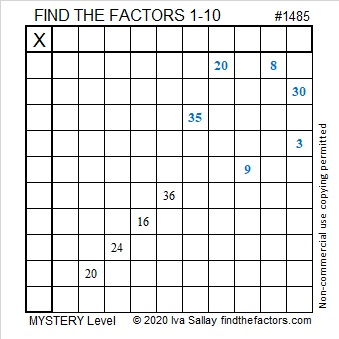### Factors of 1485:

• 1485 is a composite number.
• Prime factorization: 1485 = 3 × 3 × 3 × 5 × 11, which can be written 1485 = 3³ × 5 × 11
• 1485 has at least one exponent greater than 1 in its prime factorization so √1485 can be simplified. Taking the factor pair from the factor pair table below with the largest square number factor, we get √1485 = (√9)(√165) = 3√165
• The exponents in the prime factorization are 3,1 and 1. Adding one to each exponent and multiplying we get (3 + 1)(1 + 1) (1 + 1) = 4 × 2 × 2 = 16. Therefore 1485 has exactly 16 factors.
• The factors of 1485 are outlined with their factor pair partners in the graphic below.### More about the Number 1485:

Did you notice: (27)(55) = 1485? That means that
(54)(55)/2 = 1485 so 1485 is the 54th triangular number.

1485 is the hypotenuse of a Pythagorean triple:
891-1188-1485 which is (3-4-5) times 297.

Of the ten numbers from 1480 to 1489, four are prime numbers and have exactly 2 factors. Three of the ten numbers have exactly 16 factors, namely 1480, 1482, and 1485. No smaller set of three numbers with sixteen factors are as close together as these three are!

# 1484 A Popsicle for a Hot Summer Day

### Today’s Puzzle:

It can be tricky to eat a melting popsicle on a hot summer day. Likewise, it can be tricky to solve a level 6 puzzle even if it looks like a popsicle!  Making it was my granddaughter’s idea. We hope you enjoy it!### Factors of 1484:

If you know that 7 x 12 is 84, it isn’t hard to recognize that 1484 is divisible by 7.

• 1484 is a composite number.
• Prime factorization: 1484 = 2 × 2 × 7 × 53, which can be written 1484 = 2² × 7 × 53
• 1484 has at least one exponent greater than 1 in its prime factorization so √1484 can be simplified. Taking the factor pair from the factor pair table below with the largest square number factor, we get √1484 = (√4)(√371) = 2√371
• The exponents in the prime factorization are 2, 1, and 1. Adding one to each exponent and multiplying we get (2 + 1)(1 + 1)(1 + 1) = 3 × 2 × 2 = 12. Therefore 1484 has exactly 12 factors.
• The factors of 1484 are outlined with their factor pair partners in the graphic below.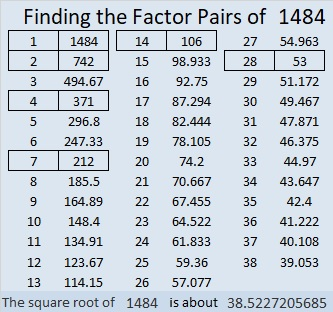### Other facts about the Number 1484:

1484 is the difference of two squares in two different ways:
372² – 370² = 1484
60² – 46² = 1484

784-1260-1484 which is 28 times (28-45-53)

# 1483 and Level 5

### Today’s Puzzle:

Some of the clues in the same row or column in this puzzle have more than one common factor. In each case, will you make the logical choice to find the puzzle’s unique solution?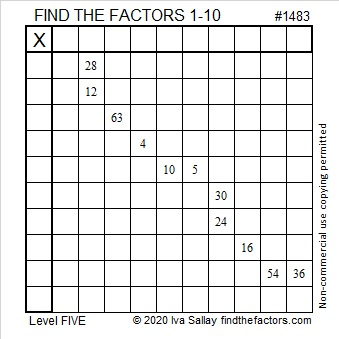### Factors of 1483:

• 1483 is a prime number.
• Prime factorization: 1483 is prime.
• 1483 has no exponents greater than 1 in its prime factorization, so √1483 cannot be simplified.
• The exponent in the prime factorization is 1. Adding one to that exponent we get (1 + 1) = 2. Therefore 1483 has exactly 2 factors.
• The factors of 1483 are outlined with their factor pair partners in the graphic below.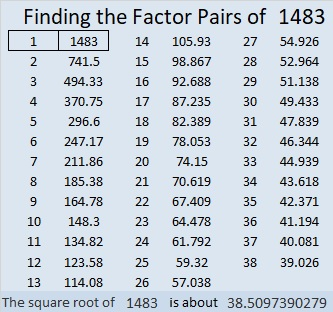How do we know that 1483 is a prime number? If 1483 were not a prime number, then it would be divisible by at least one prime number less than or equal to √1483. Since 1483 cannot be divided evenly by 2, 3, 5, 7, 11, 13, 17, 19, 23, 29 31, or 37, we know that 1483 is a prime number.

### More about the Number 1483:

1483 is the difference of two squares:
742² – 741² = 1483

The first five prime decades are listed below. 1483 is the second prime number in the fifth prime decade:# 1480 and Level 3

### Today’s Puzzle:

This is a level 3 puzzle. Start with the common factor of 21 and 14, then fill in the cells in the first column in order from top to bottom as well as the factors in the top row until you have placed all of the factors from 1 to 10 in both of those places.### Factors of 1480:

80 is divisible by 8 and 4 is even, so 1480 is divisible by 8. Here’s a little more about its factors:

• 1480 is a composite number.
• Prime factorization: 1480 = 2 × 2 × 2 × 5 × 37, which can be written 1480 = 2³ × 5 × 37
• 1480 has at least one exponent greater than 1 in its prime factorization so √1480 can be simplified. Taking the factor pair from the factor pair table below with the largest square number factor, we get √1480 = (√4)(√370) = 2√370
• The exponents in the prime factorization are 3,1 and 1. Adding one to each exponent and multiplying we get (3 + 1)(1 + 1) (1 + 1) = 4 × 2 × 2 = 16. Therefore 1480 has exactly 16 factors.
• The factors of 1480 are outlined with their factor pair partners in the graphic below.### Something else about the number 1480:

1480 is the hypotenuse of FOUR Pythagorean triples:
456-1408-1480 which is 8 times (57-176-185)
480-1400-1480 which is (12-35-37) times 40
832-1224-1480 which is 8 times (104-153-185)
888-1184-1480 which is (3-4-5) times 296

# 1479 and Level 2

### Today’s Puzzle:

Can you find the factors from 1 to 10 that will work with the clues in this puzzle to make a multiplication table?### Factors of 1479:

• 1479 is a composite number.
• Prime factorization: 1479 = 3 × 17 × 29
• 1479 has no exponents greater than 1 in its prime factorization, so √1479 cannot be simplified.
• The exponents in the prime factorization are 1, 1, and 1. Adding one to each exponent and multiplying we get (1 + 1)(1 + 1)(1 + 1) = 2 × 2 × 2 = 8. Therefore 1479 has exactly 8 factors.
• The factors of 1479 are outlined with their factor pair partners in the graphic below.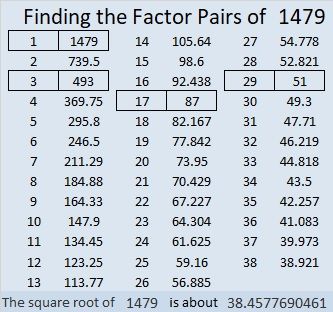### Another Fact about the Number 1479:

1479 is the hypotenuse of FOUR Pythagorean triples:
396-1425-1479 which is 3 times (132-475-493)
465-1404-1479 which is 3 times (155-468-493)
696-1305-1479 which is (8-15-17) times 87
1020-1071-1479 which is (20-21-29) times 51